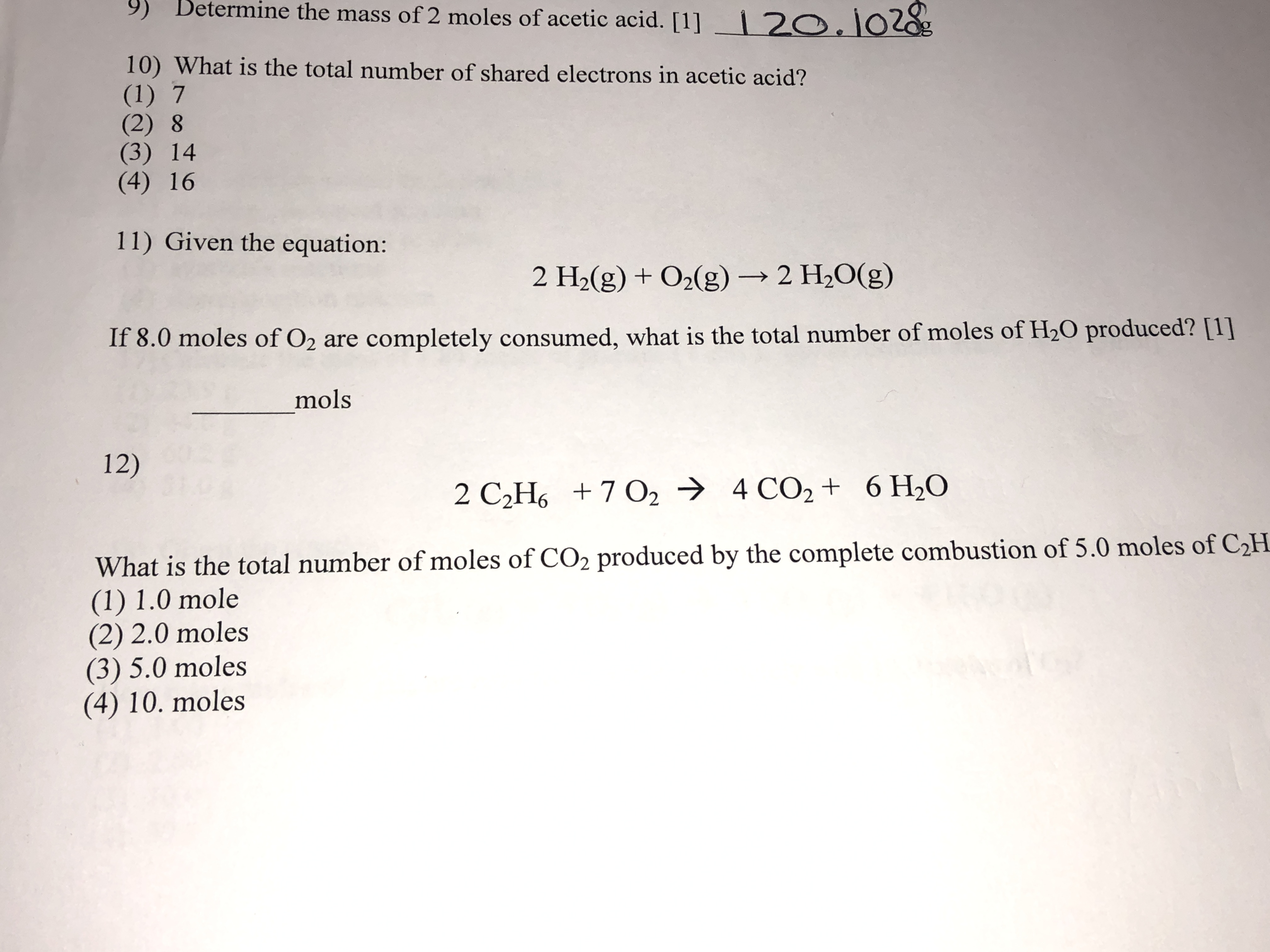# 9) Betermine the mass of 2 moles of acetic acid.  2O.102g 10) What is the total number of shared electrons in acetic acid? (1) 7 (2) 8 (3) 14 (4) 16 11) Given the equation: 2 H2(g) +02(g)2 H2O(g) If 8.0 moles of O2 are completely consumed, what is the total number of moles of H20 produced?  mols 12) 2 C2H6 7 O2 4 CO2 + 6 H20 What is the total number of moles of CO2 produced by the complete combustion of 5.0 moles of C2H (1) 1.0 mole (2) 2.0 moles (3) 5.0 moles (4) 10. moles

Question

Question 11help_outlineImage Transcriptionclose9) Betermine the mass of 2 moles of acetic acid.  2O.102g 10) What is the total number of shared electrons in acetic acid? (1) 7 (2) 8 (3) 14 (4) 16 11) Given the equation: 2 H2(g) +02(g)2 H2O(g) If 8.0 moles of O2 are completely consumed, what is the total number of moles of H20 produced?  mols 12) 2 C2H6 7 O2 4 CO2 + 6 H20 What is the total number of moles of CO2 produced by the complete combustion of 5.0 moles of C2H (1) 1.0 mole (2) 2.0 moles (3) 5.0 moles (4) 10. moles fullscreen

1 Rating

### Want to see the step-by-step answer?